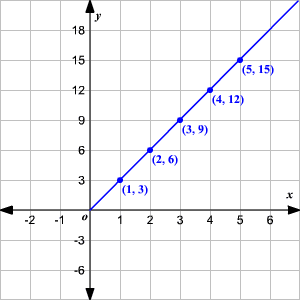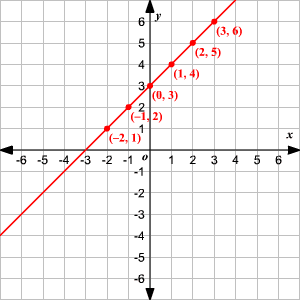# Equivalent Ratios

Suppose you have a recipe meant to feed $5$ people, but you need to feed $10$ people. How will you change the recipe? You will need to double each quantity. Similarly, if you need to feed $15$ people, you'll need to triple each quantity. But the ratios of the ingredients will be the same no matter how many people you're feeding. That is, the ratios are equivalent.

Consider the ratios $2:5$ and $4:10$ . The second ratio is obtained by multiplying each number of the first ratio by $2$ . So, they are equivalent.

If you consider two ratios as fractions, they are equivalent if their cross products are equal.

Consider the multiplication table shown.

$\begin{array}{l}1×3=3\\ 2×3=6\\ 3×3=9\\ 4×3=12\\ 5×3=15\end{array}$

The ratio of the first and last number is the same in any of the rows. That is, $\frac{1}{3}=\frac{2}{6}=\frac{3}{9}=\frac{4}{12}=\frac{5}{15}$ . The cross products of any two ratios are equal. For example, taking the first and last ratios, $1×15=5×3$ . Therefore, the ratios are equivalent.

Now, consider plotting the numbers in these ratios on a coordinate plane , with the numerators along the $x$ -axis and the denominators along the $y$ -axis. The line joining the plotted points will pass through the origin.In general, if the line joining two points on a coordinate plane passes through the origin, then the ratios of the coordinates of the points are equivalent. You can also say that the coordinates of the points are in proportion.

Example 1:

Check whether the ratios $3:4$ and $75:100$ are equivalent.

Divide each number in the second ratio by the greatest common factor , $25$ to simplify.

$\frac{75}{25}:\frac{100}{25}=3:4$

Therefore, the ratios are equivalent.

You can verify that the cross products of the ratios are equal.

Example 2:

Steve did $8$ homework problems on Saturday in $32$ minutes and $13$ problems on Sunday in $52$ minutes. Determine whether he had the same rate on both days.

Consider the ratios of the number of problems to the time taken. The ratios are $8:32$ and $13:52$ .

When you consider the corresponding fractions, both the cross products $8×52$ and $13×32$ are equal to $416$ , so the ratios are equivalent.

Therefore, Steve had the same rate on both days.

Note that if you were to plot the number of problems on $x$ -axis and time taken on $y$ -axis, the line joining the plotted points $\left(8,32\right)$ and $\left(13,52\right)$ would pass through the origin.

Example 3:

Consider the table shown.

 $x$ $y$ $3$ $6$ $2$ $5$ $1$ $4$ $0$ $3$ $-1$ $2$ $-2$ $1$

Do the pairs in each row form equivalent ratios?

Plot the points on a coordinate plane with the numbers in the first column along the $x$ -axis and those in the second column along $y$ -axis. Draw a straight line joining the plotted points.The line joining the plotted points does not pass through the origin. Therefore, the ratios are not equivalent.